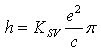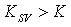## domenica 5 ottobre 2008

The Photon Double-Helicoidal Model

by studies and researches of

Oreste CaroppoAn Heuristic Model for the Photon to Explain all its Properties and its Equation E=hν :
The Electric Dipolar Double-Helicoidal Dynamic Model of the Photon

The Interpretation of the Physics Origin of the Planck ConstantThe previous photo shows the constructive interference of a beam of white light reflecting by the surface of a cd; photo by Oreste Caroppo.

The first figure represents, schematically, a photon in our model; inside the double helix, the sinusoidal curve plotted corresponds to the classical, often symbolic, representation of the photon.

Abstract

I want to propose to your attention a mechanic model for the photon. In modern physics scenery directed towards a great unification and an omni-inclusive mathematical description of the whole Universe, I think, perhaps, it needs again deepening discrete aspects of the reality. Fascinated by the photon, I thought necessary to find a photon model of its fine structure, to explain all its properties. I saw with great amazement, the energy equation of the photon,and imaging my emotion caused by a mysterious structure hidden in the photon, I began working to try to discover this elusive structure, to explain deeply the photon linear relationship between energy and frequency, confident that this new model could have opened to me a new road to explain h, Planck constant, and with it some aspects of quantum physics again dogmatic or obscure. After several years of solitary and independent studies and researches, I have the pleasure to present you my ideas and their mathematics supports. I thank my colleagues, physicists, mathematicians, professors and the other friends, who, after having read this my paper, enthusiastic, suggested me to create about it a web-page to publish it, to advertize rapidly, the scientific community all over the world, about what they call “Caroppo’s Photon Model”!

The descriptions of our model will be made in an inertial frame of reference. In our photon model, photon energy, and then its relativistic mass, we think, is divided to half between two sub-particles, separated in space, having opposite elementary charges. It is respected the electrical neutrality of the real photon, but the hypothesis of a discrete distribution of charges in it, gives us a way to understand how it is possible for the photon to carry the electromagnetic field and then, to generate the elementary electromagnetic quantum wave impulse. The two semi-photon particles of our photon model are in a dynamic equilibrium, which gives stability to the system during its propagation, creating between them a dynamic bond. In this new point of view, the generation of a matter-antimatter pair, generally electron-positron, from a photon, appears as a phenomenon implicating a breaking of the dynamic bond of the two sub-particles constituting the photon, revealing the presence in it of two particles with the same energy-mass, and with opposite elementary charges. In this heuristic photon structure, the two sub-particles, imagined punctiform, material points (at least in a first approximation), move themselves in a precise roto-translation motion along two helicoidal trajectories, two coaxial cylindrical helixes with the same step and screwing direction, and opposite between them, that is out of phase of π rad. In each moment the two semi-photon particles are on the same plane orthogonal to the common axis of the helixes, with the same distance between them, a distance equal to the common diameter of both helixes; the two photon sub-particles form, therefore, a dichotomic rigid system, an electric dipole in which the same photon would consist. The screwing angle of these helixes is 45°; the two semi-particles move themselves with a total velocity of magnitudehence, they rotate with a speed c, and they translate with an identical speed c, the speed of light, in the direction of the helix axis, therefore the photon system translates globally, along helix axis with an uniform straight line movement, of c speed, perfectly like in a real photon. The two sub-particles are tachyons; they have a superluminal speed, but their total velocity magnitude, reveals some interesting mathematic properties in special relativity equations. Radius of the helixes is such that in the time of a complete rotation, of 2π rad, the photon system translates of λ, the wavelength of the real photon of the same energy; λ is the common step of both helixes. The inverse of the time of a complete rotation, the frequency, ν, of the rotation movement, is then, the frequency of the photon. Now, in the time of a complete rotation, which is the time of a translation of the system of a wavelength, λ, effectively we have a complete rotation-fluctuation of the electric and magnetic fields of our photon model, and therefore of its associated and by it generated, electromagnetic wave! Therefore, by our model, we give a more defined description of λ and ν , wave properties of the photonic particle, that, here, we bring back to the intrinsic kinematics of the photon fine structure; a result which can recompose the traditional “wave-corpuscle dualism” in the phenomenological behaviour of the electromagnetic radiation, without the necessity of a dogmatic principle of dualism for it. This photon system, as described, has a momentum which is the same of a real photon with the same energy; identical magnitude and identical momentum direction, that of the translation motion of our system, hence of the real photon we want to describe structurally here. Our system has a spin which is exactly, that of the real photon, also with one xor the other of the two possible right-handed and left-handed screwing directions, corresponding respectively, to a vector spin parallel xor anti-parallel with the translation direction. Magnetic momentum of spin is null as in the real photon, too. Also, the total rest mass of our photonic system, which is zero, is the same of the real photon. More, we find in our model a three-dimensional photon, non punctiform, with a transversal radius linearly proportional to λ, and of the same magnitude, exactly as diffraction experiments suggest us for the real photons; however, λ, which is traditionally a longitudinal property, does not lose here, this longitudinal aspect! The magnetic Lorentz forces between the opposite charges in motion of the two semi-photon particles are null in our described system. The dynamic stability of Caroppo’s Photon Model, with the relativistic formula of Newton's second law of motion, considering the electric Coulomb force between the two photon sub-particles, gives us a surprising result, proper the equation of a linear relationship between photon energy and its frequency, as obtained in the studies of Planck and Einstein, and as verified after, in many other experiments! The discussion about the proportional constant of this equation, which must be equalled to the experimental Planck constant, h, gives us the opportunity to present a new interpretation of h. We findwhere Ksv is the magnitude of Coulomb’s constant, in Coulomb’s electrostatic interaction between the charges of the two superluminal photon sub-particles. If K is traditional Coulomb’s constant in the vacuum, we haveWe attribute the greater magnitude of Ksv , to the inertia of the vacuum, a dielectric inertia, that is the intrinsic inertia of the charged particles of the vacuum, “virtual” particles called according to modern physics; particles connected to the intrinsic energy of the empty space. For their inertia, we think they can not respond to the too much variation in space and time of the electric field connected with the superluminal passage of the internal charges of the photon, so the charges of the photon sub-particles are not screened, and they travel in a vacuum not polarized, while ever it is polarized for the electric interaction between charges in subluminal speed. This could also explain the nature of the origin of the “Fine Structure Constant”! The analyses of the electric and magnetic dynamic fields of our photon system, (that is of the elementary electromagnetic quantum wave impulse), generated by its constituting two opposite charges in motion, show exactly, in particular important straight lines, the same characteristics as orthogonalities, circular polarizations, time and space variations of their Cartesian components, which we find in the plane monochromatic electromagnetic wave in Maxwell’s theory!A theoretical model so potent that can not be ignored, although it takes us towards new revolutionary prospectives of looking at nature, certainly ever joinable with our previous scientific conquests as the history of science suggests!

Prolegomenon

In this article I propose a possible model to describe photon structure. The necessity of this model is born to understand the phenomenological photon characteristics and to solve some aspects of the quantum physics which have until now a dogmatic nature and which are covered by an intellectual fog. A photon model so astonishing that gives us the possibility to obtain the important equationby electric and dynamic intrinsic aspects of the fine structure of the photon!

For a profound depeening of this new theoretical model, I present you a complete and esaustive work about it. You will find a sequence of images of the pages of a my published pamphlet. Zoom every pages to read better.